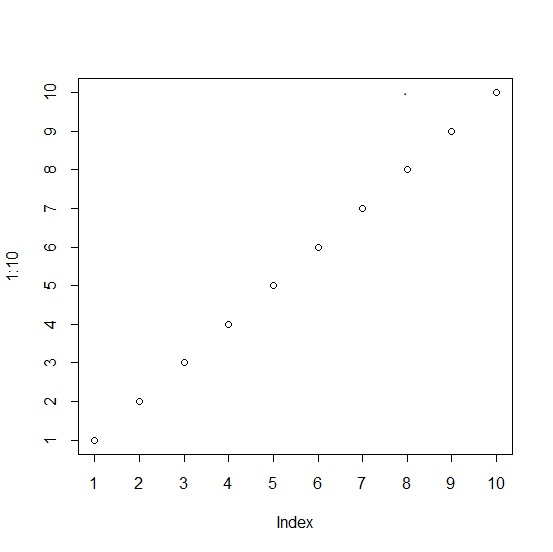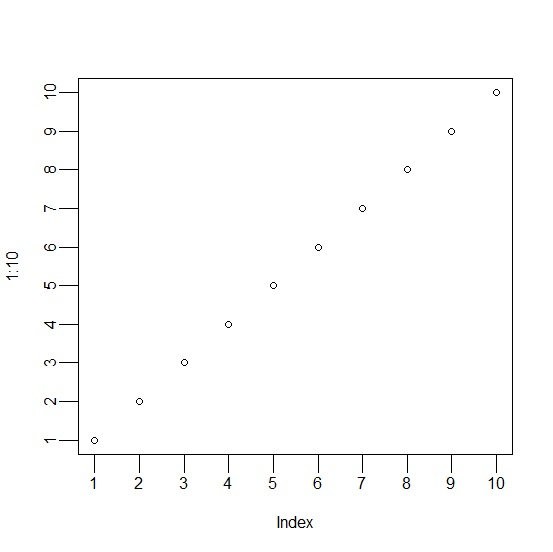# How to create a plot in base R with tick marks of larger size?

To create a plot in base R with tick marks of larger size, we can make use of axis function tck argument. The tck argument value will decide the size of the tick mark but since the ticks lie below the plot area hence the value will have a negative associated with it. Therefore, it will be like -0.05. Check out the below examples to understand how it works.

## Example

plot(1:10,axes=FALSE,frame=TRUE)
axis(1,1:10,tck=-0.02)
axis(2,1:10,tck=-0.02)

## Output## Example

plot(1:10,axes=FALSE,frame=TRUE)
axis(1,1:10,tck=-0.05)
axis(2,1:10,tck=-0.05)

## Output